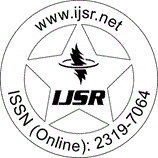International Journal of Science and Research (IJSR)
Call for Papers | Fully Refereed | Open Access | Double Blind Peer Reviewed

Research Paper | Physics Science | Sudan | Volume 3 Issue 3, March 2014

# Derivation of Einsteins Energy Equation from Maxwells Electric Wave Equation

K. G. Elgaylani  | M. D. Abd Allah  | K. M. Haroun  | M. H. Eisa  | M. A. Al Rajhi  | A. Eid

Abstract: The derivation of the equivalence of mass-energy from the principles of special relativity theory (SRT) by Einstein is arguably the most famous equation in physics. Since this derivation was published, it has been the subject of continuing controversy. In this paper, Einsteins Mass-Energy Equation will derive from Maxwells electric wave equation. In addition to that, the evaluation of photon mass can be found. The electric field wave equation for polar material from utility of Maxwells equations, beside the relations that expressed macroscopic polarization of the medium, permittivity and permeability of free space were derived.

Keywords: Einstein Equation, Maxwells Wave Equation, Photon mass, polarization, Einsteins equation

Edition: Volume 3 Issue 3, March 2014,

Pages: 686 - 690